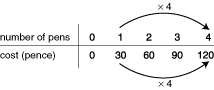اصل مواد کو چھوڑ کر آگے جائیں تلاش باکس کو چھوڑ کر آگے جائیں

Definition: proportion 1 from Merriam-Webster's Collegiate(R) Dictionary

(14c) 1 : harmonious relation of parts to each other or to the whole :balance symmetry 2 a : proper or equal share 〈each did her ⁓ of the work〉 b : quota percentage 3 : the relation of one part to another or to the whole with respect to magnitude, quantity, or degree :ratio 4 : size dimension 5 : a statement of equality between two ratios in which the first of the four terms divided by the second equals the third divided by the fourth (as in 4/2=10/5) compare extreme 1b mean 1c — in proportion : proportional 1

Summary Article: proportion
From The Hutchinson Unabridged Encyclopedia with Atlas and Weather Guide

Relation of a part to the whole (usually expressed as a fraction or percentage). In mathematics two variable quantities x and y are proportional if, for all values of x, y = kx, where k is a constant. This means that if x increases, y increases in a linear fashion.

Direct proportion If A and B are in direct proportion, then as one grows bigger the other also grows bigger by the same proportion (or ratio). For example, if B doubles then A doubles. This can be written as AB or A = kB (where k is a constant multiplier). For example, when costing a number of pens:For every extra pen bought, the cost goes up by the same amount (30p). So the cost of the pens will be proportional to the number bought.

If A is proportional to B there are two things that are true:

the multiplier rule – if A is multiplied by a value then B must be multiplied by the same value.

the graph of A against B is always a straight line through (0,0) and the gradient of the graph is the same as the ratio of A:B.

Inverse proportion If A is inversely proportional to B, then as B gets bigger A gets smaller by the same factor. For example, if B is increased by a factor of 2 (B doubles) then A decreases by a factor of 2 (A is halved). This can be written as:

A ∝ 1/B or A = k/B

For example, a group of people want to hire a minibus at a charge of £60 a day. If only 1 person uses the minibus it will cost them £60, for 2 people the cost will be £30 each, and for 3 people the cost will be £20 each. A table and graph showing the number of people and cost can be completed:cost per person ∝ 1/peoplecost per person = k/people

k = the hire of the bus (£60), so cost per person = 60/people

The shape of the graph is typical of an inversely proportional relationship.

Many laws of science relate quantities that are proportional (for example, Boyle's law).

essays

Calculating with proportion

Proportional Representation – Using Proportion in Paper Sizes

The Hole in the Ground – Using Inverse Proportion

Maths for Morons Like Us© RM, 2018. All rights reserved.

### متعلقہ کریڈو مضامین

##### Full text Article Proportion
Bloomsbury Guide to Human Thought

Proportion is a mathematical concept, important in all the visual arts , but most significant in the field of architecture , where it is...

##### Full text Article proportion
The Bloomsbury Guide to Art

In, for example, any picture, sculpture or building, the ratio between the constituent parts one to another, or any of the parts to the whole....

##### Full text Article proportion
Philip's Encyclopedia

Mathematical relation of equality between two ratios, having the form a/b = c/d. A continued proportion is a group of three or more quantities,...

کریڈو سے مزید دیکھیں